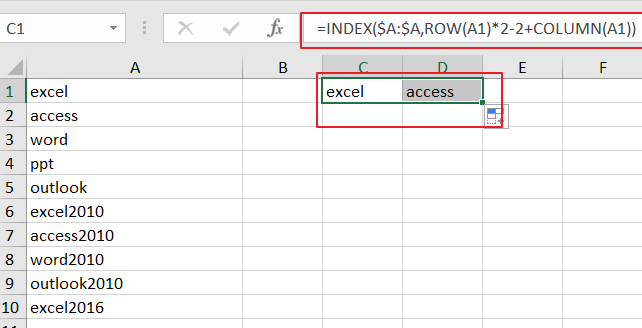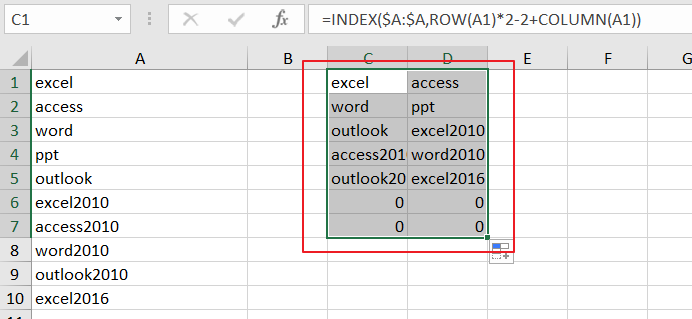# How To Transpose Every N Rows of Data into Muliptle Columns in Excel

This post will guide you how to transpose data from rows to column with a formula in Excel. How do I transpose every N rows from one column to multiple columns in Excel.

Assuming that you have a list of data in range A1:A10 in column A, and you want to transpose every 2 rows from column A to Mulitple columns. for example, you want to transpose range A1:A2 to C1:D1, A3:A4 to C2:D2. How to do it. And you can user an formula based on the INDEX function, the Row function and the Column Function. Like as below:

```=INDEX(\$A:\$A,ROW(A1)*2-2+COLUMN(A1))
```

You need to type this formula into cell C1, and press `Enter` key on your keyboard, and then drag the AutoFill Handle to CEll D1.Then you need to drag the AutoFill Handle in cell D1 down to other cells until` value 0` is displayed in cells.### Related Functions

• Excel INDEX function
The Excel INDEX function returns a value from a table based on the index (row number and column number)The INDEX function is a build-in function in Microsoft Excel and it is categorized as a Lookup and Reference Function.The syntax of the INDEX function is as below:= INDEX (array, row_num,[column_num])…
• Excel ROW function
The Excel ROW function returns the row number of a cell reference.The ROW function is a build-in function in Microsoft Excel and it is categorized as a Lookup and Reference Function.The syntax of the ROW function is as below:= ROW ([reference])….
• Excel COLUMN function
The Excel COLUMN function returns the first column number of the given cell reference.The syntax of the COLUMN function is as below:=COLUMN ([reference])….